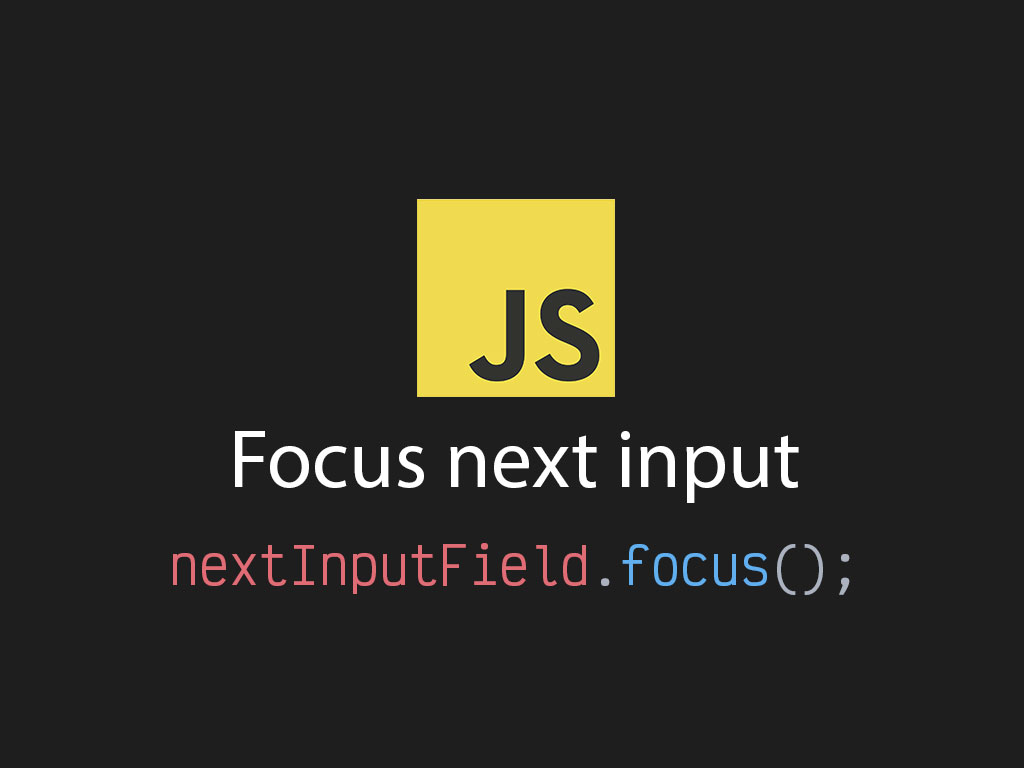# Focus next input in ReactI recently had to create a form that had a social security number field. Here was the markup.

``````
const SSNField = () => {

return (
<>
<input
type="text"
name="ssn-1"
maxLength={3} />
<input
type="text"
name="ssn-2"
maxLength={2} />
<input
type="text"
name="ssn-3"
maxLength={4} />
</>
);
};
``````

If you ever seen one of these fields, they’re usually made up of 3 `<input>` elements that make up 1 value.

Each field has a max length value, and have been given the property, `name`.

With that current user experience, the user types in the input field and gets stopped from entering anymore characters because of the `maxLength` property.

The user than has to manually click on the next field to continue. That’s really annoying.

A better user experience is to automatically focus to the next input field when they hit the max character length.

Here’s how I did it.

## Focusing the next input after reaching the max length

The objective is to find the next input html element, and set that as the new focus point.

Only if the user has reached the max character length in the input field.

``````
// Number of input fields that make up SSN
const numOfFields = 3;

const handleChange = e => {
const { maxLength, value, name } = e.target;
const [fieldName, fieldIndex] = name.split("-");

// Check if they hit the max character length
if (value.length >= maxLength) {
// Check if it's not the last input field
if (parseInt(fieldIndex, 10) < 3) {
// Get the next input field
const nextSibling = document.querySelector(
`input[name=ssn-\${parseInt(fieldIndex, 10) + 1}]`
);

// If found, focus the next field
if (nextSibling !== null) {
nextSibling.focus();
}
}
}
}
``````

Now that the `onChange` handler function has been created, it’s time to attach it to the input elements.

``````
const SSNField = () => {

return (
<>
<input
type="text"
name="ssn-1"
maxLength={3}
onChange={handleChange} />
<input
type="text"
name="ssn-2"
maxLength={2}
onChange={handleChange} />
<input
type="text"
name="ssn-3"
maxLength={4}
onChange={handleChange} />
</>
);
};
``````

## Full code with React useState

Here’s a bonus with `React.useState()`.

``````
// Number of input fields that make up SSN
const numOfFields = 3;

const useSSNFields = () => {
const [ssnValues, setValue] = React.useState({
ssn1: "",
ssn2: "",
ssn3: ""
});

return {
handleChange: e => {
const { maxLength, value, name } = e.target;
const [fieldName, fieldIndex] = name.split("-");

// Check if they hit the max character length
if (value.length >= maxLength) {
// Check if it's not the last input field
if (parseInt(fieldIndex, 10) < 3) {
// Get the next input field
const nextSibling = document.querySelector(
`input[name=ssn-\${parseInt(fieldIndex, 10) + 1}]`
);

// If found, focus the next field
if (nextSibling !== null) {
nextSibling.focus();
}
}
}

setValue({
...value,
[`ssn\${fieldIndex}`]: value
});
}
};
};

const SSNField = () => {
const { handleChange } = useSSNFields();

return (
<>
<input
type="text"
name="ssn-1"
maxLength={3}
onChange={handleChange} />
<input
type="text"
name="ssn-2"
maxLength={2}
onChange={handleChange} />
<input
type="text"
name="ssn-3"
maxLength={4}
onChange={handleChange} />
</>
);
};
``````

I like to tweet about React and post helpful code snippets. Follow me there if you would like some too!